Physical States and Properties

# Properties of Gases

by Megan Cartwright, Ph.D., Anthony Carpi, Ph.D.

Since 1924, the Macy’s Thanksgiving Day Parade has wound through 2.5 miles of New York City once a year. More than three million people gather to enjoy the boisterous marching bands, laugh at the hundreds of clowns, and gawk at the gigantic balloons floating above the parade. Designed to look like cartoon characters such as Snoopy (Figure 1), each massive, helium-filled balloon needs 90 handlers to safely tow it through the parade.

At first glance, the helium gas inside these balloons seems very different from the air outside them. For one thing, the balloons would be a lot less impressive if filled with air—instead of floating above the parade, they would be dragged along the ground. For another, while everyone enjoying the parade must breathe air to survive, they should only breathe helium if they want a squeaky voice. Even on a molecular level, air and helium are different: Air is a mixture of nitrogen, oxygen, and other gases, while helium is a single gas.

But helium and air have many things in common with each other, and even with substances like deadly carbon monoxide and flammable hydrogen. At standard temperature and pressure, these substances are all gases, one of the common states of matter (see our module States of Matter for more information). All gases share common physical properties. Like liquids, gases freely flow to fill the container they are in. But while liquids have a defined volume, gases have neither a defined volume nor shape. And unlike liquids and solids, gases are highly compressible.

These common properties relate to a unique characteristic of gases: Gas molecules are incredibly far apart and rarely interact with each other. In solids, the attractive and repulsive forces between molecules—the intermolecular forces—are so strong they lock the solid into a fixed shape and size, as discussed in our Properties of Solids module. In liquids, the intermolecular forces are weaker, and liquid molecules can move around each other. But a liquid's molecules are still close enough that intermolecular forces affect nearby molecules (see our Properties of Liquids module). A gas’s molecules are so far apart that the intermolecular forces are negligible.

Because gas molecules don’t interact with one another, gases don’t exist as different types like liquids and solids do. The different types of liquids and solids (such as molecular and network solids) have properties that reflect the unique ways their molecules interact. As a result, all gases share some common behaviors. We can understand how any gas—whether it’s helium or carbon monoxide—behaves by understanding the laws governing gas behavior.

## The gas laws

Over the past four centuries, scientists have performed many experiments to understand the common behaviors of gases. They have observed that a gas's physical condition—its state—depends on four variables: pressure (P), volume (V), temperature (T), and amount (n, in moles; see our module The Mole: Its History and Use for more information). The relationships between these variables are now known as the gas laws, which describe our current knowledge about how gases behave on a macroscopic level.

But the relationships behind the gas laws weren't obvious at first—they were uncovered by many scientists examining and testing their ideas about gases over many years.

### Gas pressure

We now understand that air is a gas made of physical molecules (for more information, see our module on Atomic Theory). As these molecules move about inside a container, they exert force—known as pressure—on the container when they ricochet off its walls. Thanks to this behavior, we can inflate car tires, rubber rafts, and Macy’s Day Parade balloons with gases. However, the idea that air is a substance made of molecules that exert pressure would have been a strange idea to scientists before the 17th century. Along with fire, water, and earth, air was generally considered a fundamental substance, and not one made up of other things. (For more information on this concept, see our Early Ideas about Matter: From Democritus to Dalton module.)

However, in 1644, the Italian mathematician and physicist Evangelista Torricelli proposed a strange idea. In a letter to a fellow mathematician, Torricelli described how he had filled a long glass tube full of mercury. When he sealed one end and inverted the tube into a basin, only some mercury flowed into the basin. The rest of the mercury stayed up in the tube, filling it to a height of approximately 29 inches or 73.6 centimeters (Figure 2). Torricelli proposed that it was the weight of air that pressed down on the mercury in the basin that forced the liquid up into the tube (this was one of the first known devices that we now call barometers).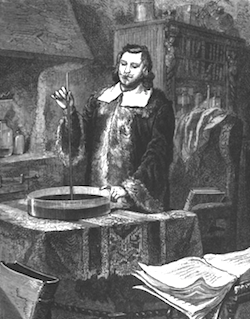Figure 2: Evangelista Torricelli experimenting with a tube of mercury and inventing the barometer. (Image from L'Atmosphere published in 1873.)

The Jesuit scientist Franciscus Linus had a different idea about what was holding the mercury up in the tube. He proposed that the mercury was being pulled up by "funiculus"—an invisible substance that materialized to prevent a vacuum forming between the mercury and sealed tube top.

The British scientist Robert Boyle disagreed, and came up with an experiment to disprove Linus’s funiculus idea. Working with the English physicist Robert Hooke, Boyle made a long glass tube that was curved like a cane, and he sealed off the short leg of the cane. Resting the curve on the ground so that both ends pointed up, Boyle poured in just enough mercury so that the silver liquid filled the curve and rose to the same height in each leg. This trapped air inside the sealed short leg.

Boyle then poured in more mercury and observed with “delight and satisfaction” that the trapped air in the sealed short end supported a 29-inch-tall (73.6 cm) column of mercury in the long leg—the same height that the mercury reached in Torricelli’s barometer. However, because there was no cap on the long leg, there could be no funiculus pulling up the extra mercury. Boyle reasoned that it must be the trapped air’s pressure (which he called “spring”) that pushed the mercury up those 29 inches.

To understand more about air’s pressure, Boyle poured more mercury into the curved tube. He recorded the height of the mercury column in the long leg, and the height of the trapped air in the short leg. After repeating these steps many times, Boyle was able to observe the relationship between the height of the trapped air—its volume—and the height of the growing mercury column—an indicator of the pressure in the tube. Even though scientists in Boyle’s time generally didn’t graph data, we can best see this relationship by graphing Boyle’s data (Figure 3).Figure 3: The plot of Robert Doyle's data that he recorded during his experiment on mercury and trapped air in glass tubes. image © Krishnavedala

### Boyle's law

Boyle’s data showed that when air was squeezed to half its original volume, it doubled its pressure. In 1661, Boyle published his conclusion that air’s volume was inversely related to its pressure. This observation about air’s behavior—and therefore, gas behavior—is a critical part of what we now call Boyle’s law.

Boyle’s law states that so long as temperature is kept constant, the volume (V) of a fixed amount of gas is inversely proportional to its pressure (P) (Figure 4):

Equation 1a

$$V ∝ 1 P$$Figure 4: Boyle's law states that so long as temperature is kept constant, the volume of a fixed amount of gas is inversely proportional to the pressure placed on the gas.

Boyle’s law can also be written as:

Equation 1b

$$V × P = constant$$

For a fixed amount of gas at a fixed temperature, this constant will be the same, even if the gas’s pressure and volume change from (P1, V1) to (P2, V2), because volume decreases as pressure increases. Therefore, P1 x V1 must equal the constant, and P2 x V2 must also equal the constant. Because they both equal the same constant, the gas’s pressure and volume under two different conditions are related like this:

Equation 2

$$P 1 × V 1 = P 2 × V 2$$

Going back to that helium balloon shaped like Snoopy, Boyle’s law means that if you took the balloon deep under the ocean, poor Snoopy would shrivel because the pressure is very high and the helium would significantly decrease in volume. And if you took the balloon to the top of Mount Everest, Snoopy would get even bigger (and might even pop!) because the atmospheric pressure is low and the helium would increase in volume.

Comprehension Checkpoint

Which two variables describing a gas's state are inversely related, according to Boyle's law?

### Charles's law

More than a century after Boyle’s work, scientists had figured out another important behavior of air: Air expands when heated, and hot air rises above cooler air. Taking advantage of this air behavior, the French brothers Joseph-Michel and Jacques-Étienne Montgolfier launched the first successful hot-air balloon in Paris in 1783.

The Montgolfiers’ balloon fascinated Jacques-Alexandre-César Charles, a self-taught French scientist interested in aeronautics. He had an idea about how to make an even better balloon. From his familiarity with contemporary chemistry research, Charles knew that hydrogen was much lighter than air. In 1783, Charles built and launched the first hydrogen balloon (see Figure 4 for an example of the balloon launch). Later that year, he became the first human to ride in a hydrogen balloon, which reached almost 10,000 feet above Earth.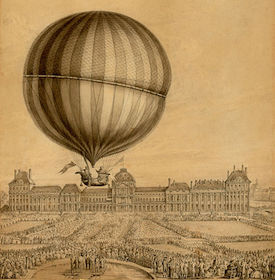Figure 4: Jacques Charles and Nicolas Marie-Noel Robert standing in their hydrogen-filled balloon waving flags, beginning their ascent in Paris. Thousands of spectators are gathered in the foreground to witness the first manned gas balloon flight.

Charles was very fortunate that he survived riding in a hydrogen balloon: On May 6, 1937, 36 people died when the Hindenburg airship, a dirigible filled with flammable hydrogen, caught fire and crashed to the ground. The airship’s flammable hydrogen gas may have been ignited by a lightning bolt or spark from static electricity, and the fire spread explosively throughout the ship in a matter of seconds.

While Charles never rode a balloon again, he remained fascinated with the gases inside balloons. In 1787, Charles conducted experiments comparing how balloons filled with different gases behaved when heated. Intriguingly, he found that balloons filled with gases as different as oxygen, hydrogen, and nitrogen expanded the same amount when their temperatures were heated from 0 to 80°C. However, Charles did not publish his findings. We only know about his experiments because they were mentioned in the work of another French chemist and balloonist, Joseph-Louis Gay-Lussac.

In 1802, Gay-Lussac published his results from similar experiments comparing nine different gases. Like Charles, Gay-Lussac concluded that it was a common property of all gases to increase their volume the same amount when their temperature was increased by the same degree. Gay-Lussac graciously gave Charles credit for first observing this common gas behavior.

This relationship between a gas’s volume (V) and absolute temperature (T, in Kelvin; to learn more about absolute temperature, see our Temperature module) is now known as Charles’s law. Charles’s law states that when pressure is kept constant, a fixed amount of gas linearly increases its volume as its temperature increases (Figure 5):

Equation 3a

$$V ∝ T$$Figure 5: Charles's Law states that when pressure is kept constant, a fixed amount of gas linearly increases its volume as its temperature increases.

Charles’s law can also be understood as:

Equation 3b

$$V T ∝ constant$$

For a fixed amount of gas at a fixed pressure, this constant will be the same, even if the gas’s volume and temperature change from (V1, T1) to (V2, T2). Therefore, V1/T1 must equal the constant, and V2/T2 must also equal the constant. As a result, the gas’s temperature and volume under different conditions are related like this:

Equation 4

$$V 1 T 1 = V 2 T 2$$

This means that if we took the Snoopy balloon to the North Pole, the balloon would shrink as the helium cooled and decreased in volume. However, if we took the balloon to a hot tropical island and the helium’s temperature increased, the helium would increase in volume, expanding the balloon.

Comprehension Checkpoint

When different gases are heated up by the same number of degrees, their volume will

After his work on Charles’s law, Gay-Lussac focused on figuring out how gases reacted and combined. In 1808, he observed that many gases combined their volumes in simple, whole-number ratios. While we understand now that volumes of gases combine in whole-number ratios because that is how the gas molecules react, Gay-Lussac didn’t suggest this explanation. This was probably because the idea of whole-number molecular combinations had only recently been proposed by John Dalton, who was Gay-Lussac’s scientific rival. (For further exploration of how gas molecules react, see our Chemical Equations module).

It was the Italian mathematician Amedeo Avogadro who realized that Dalton’s and Gay-Lussac’s ideas complemented each another. Gay-Lussac’s claim that gas volumes combined in whole-number ratios resembled Dalton’s claim that atoms combined in whole-number ratios to form molecules. Avogadro reasoned that a gas’s volume must then be related to the number of its molecules. In 1811, Avogadro published his hypothesis that equal volumes of different gases have an equal number of molecules.

Avogadro’s hypothesis was ground-breaking though largely overlooked. The mathematician rarely interacted with other scientists, and he published his hypothesis with mathematical expressions that were unfamiliar to chemists. He also didn’t publish experimental data to support his hypothesis.

It was 47 years before Avogadro’s hypothesis would be broadly recognized. In 1858, a former student of Avogadro, the Italian chemist Stanislao Cannizzaro, published an influential work on atomic theory. This work drew on Avogadro’s hypothesis and presented experimental data supporting the hypothesis.

Avogadro’s law is based off of Avogadro’s hypothesis. Avogadro’s law states that at a constant pressure and temperature, a gas’s volume (V) is directly proportional to the number of molecules (n, in moles) (Figure 6):

Equation 5

$$V ∝ n$$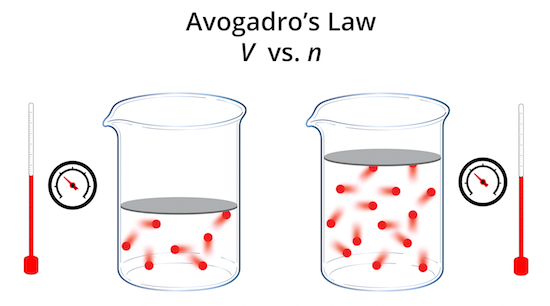Figure 6: Avogadro's Law states that at a constant pressure and temperature, a gas's volume is directly proportional to the number of molecules.

We know that a Snoopy balloon filled with helium will float above the parade, while the same balloon filled with air will drag along the ground. While helium and air are different in many ways, Avogadro’s law means that if we compared the number of helium molecules and the number of air molecules needed to inflate the same Snoopy balloon, we would find that the numbers are the same.

Comprehension Checkpoint

According to Avogadro's law, 1 liter of toxic carbon monoxide gas and 1 liter of flammable hydrogen gas both have the same:

## Ideal gas equation

Because gases have common behaviors described by the gas laws, we can understand and predict the behavior of real gases through the concept of an ideal gas—a theoretical, idealized gas that always behaves according to the ideal gas equation.

The ideal gas equation is derived from the gas laws. This equation describes the relationships between all of the variables examined in the gas laws: pressure (P), volume (V), amount (n, in moles), and absolute temperature (T, in Kelvins). Along with the gas constant, R, these variables combine into the ideal gas equation:

Equation 6

$$P V = n R T$$

Using the ideal gas equation, we can solve for any one of the unknown variables, so long as we know the others. The value for R depends on the units used for the other variables (Table 2).

Table 2: Values of the gas constant R for different units.
Units R's Value
cal K-1 mol-1 1.9872
J K-1 mol-1 8.3145
L atm K-1 mol-1 0.0821
L Torr K-1 mol-1 62.364
Pa m3 K-1 mol-1 8.3145

The ideal gas law assumes that an ideal gas’s molecules have no volume, and experience no intermolecular attraction or repulsion. But real gas molecules do have finite volume and often have some (very small) interactions with each other. Nonetheless, the behavior and state of a real gas can often be predicted from the ideal gas equation, especially at standard temperature and pressure. Under most conditions the difference between a real gas’s behavior and an ideal gas’s behavior is so small that we can use the ideal gas equation for real gases.

We’ll explore several conditions where a real gas behaves differently from an ideal gas at the end of this module.

Comprehension Checkpoint

Ideal gas molecules have

### Combined gas law

The ideal gas law is also useful in situations where the amount, n, of gas is fixed, but its pressure, volume, and temperature change. Using the ideal gas law, we can relate the value of these three variables under different conditions. To do this, we have to first rearrange the ideal gas equation so that the three changing variables equal nR:

Equation 7a

$$P V = n R T$$

Equation 7b

$$P V T = n R ∝ constant$$

This relationship is referred to as the combined gas law. Because nR is a constant, we can relate the initial (P1, V1, T1) and the final conditions (P2, V2, T2) of a gas like this:

Equation 8

$$P 1 V 1 T 1 = P 2 V 2 T 2$$

### Sample problem using the ideal gas equation

From 1987 to 2012, air bags (Figure 6) saved almost 37,000 American lives in car crashes. Air bags save lives because when a car stops hard during a crash, a sensor triggers a chemical reaction to generate nitrogen gas. The nitrogen gas inflates the air bag, which essentially forms a big pillow between the driver and the steering wheel. The pillow spreads out the force of the crash’s impact, helping reduce the severity of the driver’s injuries.

For an air bag to work, it has to inflate full of nitrogen incredibly fast—within 40 milliseconds of the collision. For a 60-liter cylindrical air bag to work properly, the nitrogen gas has to reach a pressure of 2.37 atm. At 25°C, how many moles of nitrogen gas are needed to pressurize the air bag?

We can figure this out using the ideal gas equation. First, we list the values we know, and convert them so they have the same units as the gas constant, R (0.0821 L-atm/mol-K).

$$P = 2.37 atm$$

$$V = 60 L$$

$$T = 25 ∘ C = ( 25 + 273 ) K = 298 K$$

Next, we rearrange the ideal gas equation to solve for the number of moles, n:

$$P V = n R T$$

$$n = P V R T$$

Finally, we solve for the number of moles of nitrogen gas to pressurize the air bag:

$$n = ( 2.37 atm ) × ( 60 L ) ( 0.0821 L ⋅ atm mol ⋅ K ) × ( 298 K )$$

$$n = 5.81 moles$$

## Deviations from ideal gas behavior

### Low temperature

Real gases frequently deviate from ideal gases when their temperature gets low, especially when it’s close to where the gas would undergo a phase change into its liquid form. When a gas’s temperature decreases, its molecules move slower. These slower molecules are less able to overcome even the weak intermolecular forces in a gas.

This means that when a gas molecule is about to strike the wall of a container, the very small attraction it experiences for nearby gas molecules reduces its impact and the pressure it exerts on the container. Therefore, a real gas at a low temperature exerts a lower pressure in a container (Figure 7), compared to an ideal gas.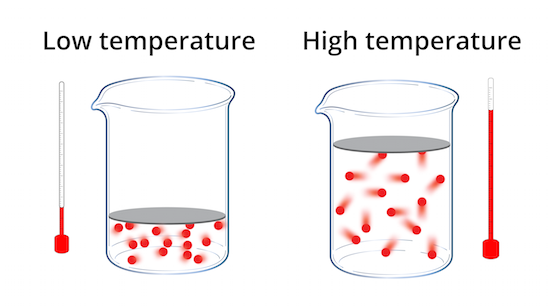Figure 7: A real gas at low temperature exerts a lower pressure than predicted due to the attraction between molecules of the gas.

### High pressure

Under high pressure, a real gas frequently deviates from ideal gases because the real gas’s molecules do have volume, and do attract each other.

When a real gas is under high pressure, its molecules are forced into a smaller volume. This smaller volume reduces the amount of free space the molecules have to move around (Figure 8). The amount of space a gas’s molecules take up compared to the total space in the container—the molecules’ relative volume—gets bigger.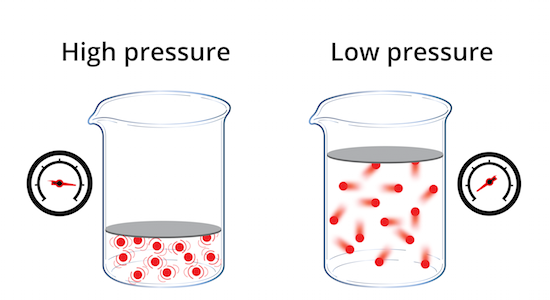Figure 8: Under high pressure, a real gas has a larger volume than predicted due to the volume of the molecules it contains.

This means that under high pressures a real gas has a higher volume than an ideal gas, because an ideal gas’s molecules have no volume.

Furthermore, when a real gas’s molecules are crowded close together, intermolecular forces can have more influence on the molecules’ behavior. The attractive intermolecular forces draw molecules towards each other, which slows down the molecules and reduces their impact on a container’s walls. Therefore, when it’s under high pressure, a real gas has a slightly lower pressure than an ideal gas.

Comprehension Checkpoint

At low temperatures, an ideal gas in a container exerts _____ than a real gas.

## Conclusion

The characteristics of gases affect many important things, from Earth’s atmosphere to air bags to how we breathe. Between breaths, the air pressure inside our lungs is the same as the atmospheric pressure around us. When we inhale and use our rib cage and diaphragm to expand our lungs’ volume, the air pressure decreases and external pressure forces air inside our lungs until the pressures are the same again—thereby filling our lungs with the oxygen we need to survive.

In this module, we have focused on the common properties of gases, and explored how these properties relate to a common set of behaviors called the gas laws. We have also gained an understanding of the ideal gas equation, and when this equation can—and cannot—be used to predict the behavior of real gases. In other modules, we examine the properties of solid and liquid states of matter, and further explore molecular explanations for gas behavior with kinetic molecular theory.

### Summary

This module describes the properties of gases and explores how these properties relate to a common set of behaviors called the gas laws. With a focus on Boyle’s Law, Charles’s Law, and Avogadro’s Law, an overview of 400 years of research shows the development of our understanding of gas behavior. The module presents the ideal gas equation and explains when this equation can—and cannot—be used to predict the behavior of real gases.

### Key Concepts

• Unlike solids or liquids, the molecules in a gas are very far apart and rarely interact with each other, which is why gases made out of different molecules share similar behaviors.

• The gas laws describe the relationships between a gas's temperature, pressure, volume, and amount. These laws were identified in experiments performed by multiple scientists over four centuries.

• Because gases share common behaviors, the behavior of a real gas at a given pressure (P), absolute temperature (T), volume (V), and amount (n, in moles) can often be predicted by the ideal gas equation, PV = nRT, which perfectly describes the behavior of an idealized gas.

• The behavior of real gases deviates from ideal gases at very low temperatures and high pressures.

• ##### References
• Ashkenazi, G., James, S.G., & Jason, D.H. (2008). Similarity and difference in the behavior of gases: An interactive demonstration. Journal of Chemical Education, 85(1): 72.

• Bell, W.L. (1990). Chemistry of air bags. Journal of Chemical Education, 67(1): 61.
• Brush, S. (1999). Gadflies and geniuses in the history of gas theory. Synthese, 119(1): 11-43.
• Cornely-Moss, K. (1995). Kinetic theory of gases. Journal of Chemical Education, 72(8): 715.
• Crane, H.R. (1985). The air bag: An exercise in Newton’s laws. The Physics Teacher, 23(9): 576-594.
• Criswell, B. (2008). Teaching Avogadro's hypothesis and helping students to see the world differently. Journal of Chemical Education, 85(10): 1372.
• Gay-Lussac, J.L. (1802). The expansion of gases by heat. Annales de Chimie, 43.
• Giunta, C.J. (2001). Using history to teach scientific method: The role of errors. Journal of Chemical Education, 78(5): 623.
• Gough, J.B. (1979). Charles the Obscure. Isis, 70(4): 576-579.
• Howard, I.K. (2001). S is for entropy. U is for energy. What was Clausius thinking? Journal of Chemical Education, 78(4): 505.
• Jensen, W.B. (2003). The universal gas constant R. Journal of Chemical Education, 80(7): 731.
• -----. (2007). How and when did Avogadro's name become associated with Avogadro's Number? Journal of Chemical Education, 84(2): 223.
• Kauffman, G.B. (1991). Sunto di un curso di filosofia chimica (Cannizzaro, Stanislao). Journal of Chemical Education, 68(10): A266.
• Laugier, A., & Garai J. (2007). Derivation of the Ideal Gas Law. Journal of Chemical Education, 84(11): 1832.
• Lipeles, E.S. (1983). The chemical contributions of Amadeo Avogadro. Journal of Chemical Education, 60(2): 127.
• Madlung, A. (1996). The chemistry behind the air bag: High tech in first-year chemistry. Journal of Chemical Education, 73(4): 347.
• Neville, R.G. (1962). The discovery of Boyle's Law, 1661-62. Journal of Chemical Education, 39(7): 356.
• Partington, J. R. (1950). J L. Gay-Lussac (1778-1850). Nature 165(4201): 708.
• Szabadváry, F. (1978). Joseph Louis Gay-Lussac (1778-1850) and analytical chemistry. Talanta, 25(11-12): 611.
• West, J.B. (1999). The original presentation of Boyle’s Law. Journal of Applied Physiology, 87(4): 1543-1545.
• -----. (2005). Robert Boyle’s landmark book of 1660 with the first experiments on rarified air. Journal of Applied Physiology, 98(1): 31-39.
• -----. (2014). Robert Hooke: early respiratory physiologist, polymath, and mechanical genius. Physiology (Bethesda), 29(4): 222-233.
• Whitaker, R.D. (1979). The early development of kinetic theory. Journal of Chemical Education, 56(5): 315.

Megan Cartwright, Ph.D., Anthony Carpi, Ph.D. “Properties of Gases” Visionlearning Vol. CHE-3 (9), 2016.

Top